# 深度学习第21讲：迁移学习的基本原理和实践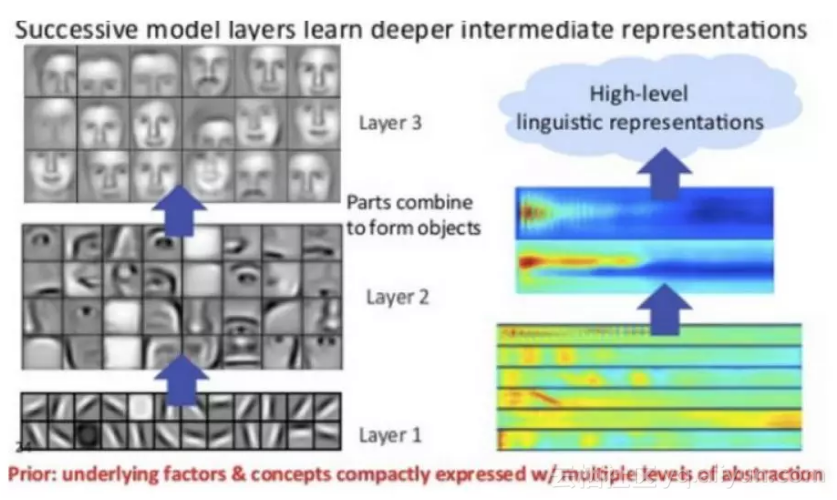keras 为我们提供了经典网络在 ImageNet 上为我们训练好的预训练模型，预训练模型的基本信息如下表所示：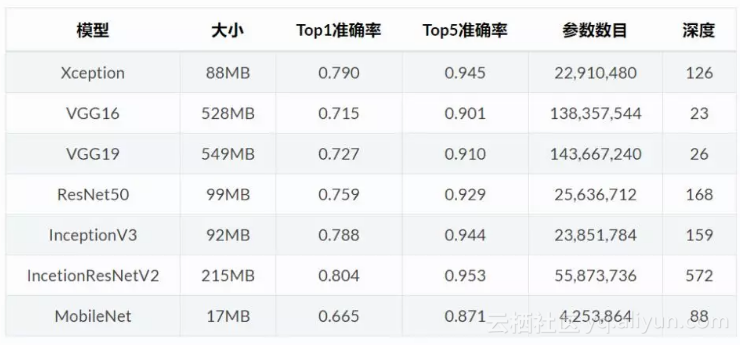from keras.models import Model
from keras.layers import Dense, Flatten, Dropout
from keras import datasets
from keras.applications.vgg16 import VGG16
from keras.optimizers import SGD
from keras.datasets import mnist
import numpy as npimport cv2
# 查看 VGG16 预训练模型的基本信息
model_vgg = VGG16(include_top=False, weights='imagenet', input_shape=(224,224,3))
model = Flatten(name='Flatten')(model_vgg.output)
model= Dense(10, activation='softmax')(model)
model_vgg_mnist = Model(inputs=model_vgg.input, outputs=model, name='vgg16')
model_vgg_mnist.summary()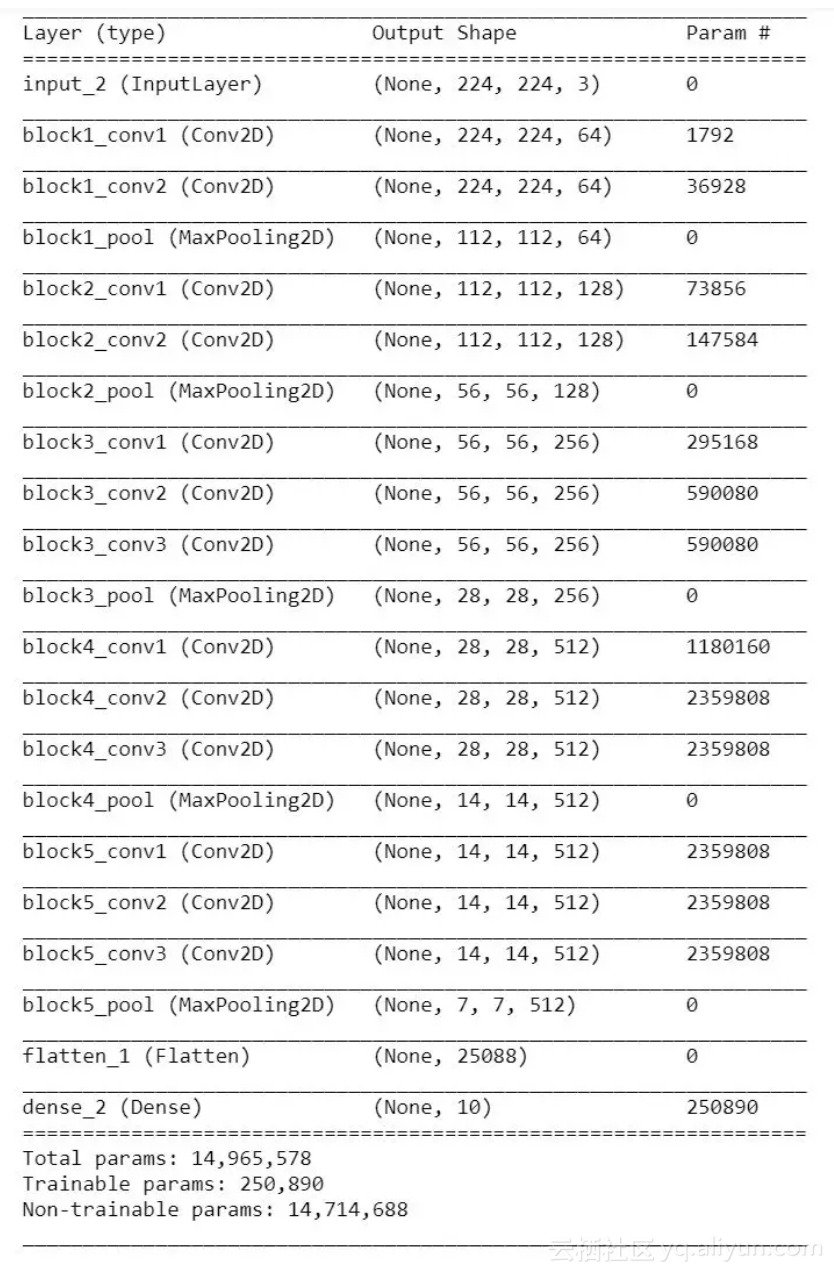model_vgg = VGG16(include_top=False, weights='imagenet', input_shape=(224, 224, 3))
for layers in model_vgg.layers:
layers.trainable = Falsemodel = Flatten()(model_vgg.output)
model = Dense(10, activation='softmax')(model)
model_vgg_mnist_pretrain = Model(inputs=model_vgg.input, outputs=model, name='vgg16_pretrain')
sgd = SGD(lr=0.05, decay=1e-5)
model_vgg_mnist_pretrain.compile(optimizer=sgd, loss='categorical_crossentropy',
metrics=['accuracy'])

X_train = [cv2.cvtColor(cv2.resize(i, (img, img)), cv2.COLOR_GRAY2BGR) for i in X_train]
X_train = np.concatenate([arr[np.newaxis] for arr in X_train]).astype('float32')
X_test = [cv2.cvtColor(cv2.resize(i, (img, img)), cv2.COLOR_GRAY2BGR) for i in X_test ]
X_test = np.concatenate([arr[np.newaxis] for arr in X_test] ).astype('float32')

X_train /= X_train/255X_test /= X_test/255
np.where(X_train != 0)

def train_y(y):
y_one = np.zeros(10)
y_one[y] = 1
y_train_one = np.array([train_y(y_train[i]) for i in range(len(y_train))])
return y_one
y_test_one = np.array([train_y(y_test [i]) for i in range(len(y_test ))])
model_vgg_mnist_pretrain.fit(X_train, y_train_one, validation_data=(X_test, y_test_one),
epochs=10, batch_size=128)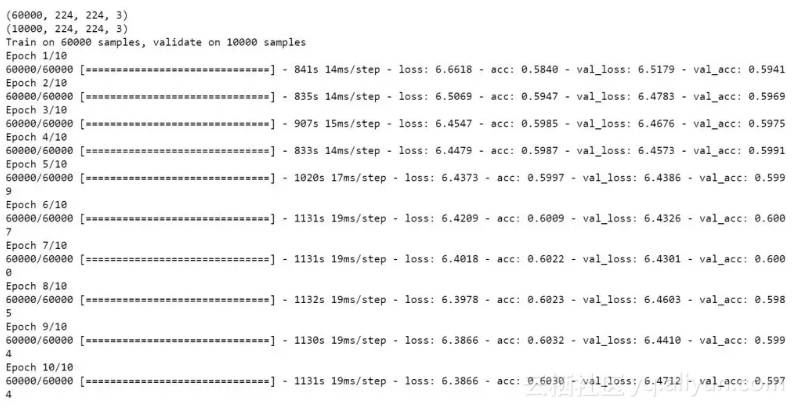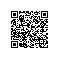Python爱好者使用钉钉扫一扫加入圈子
+ 订阅